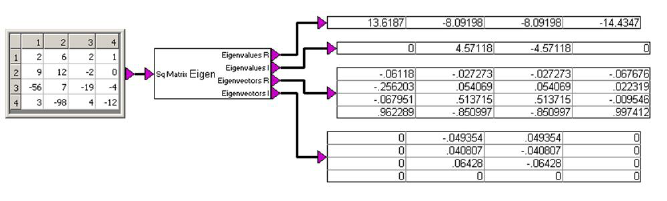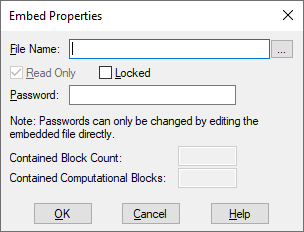## *Block Category: Arithmetic

Inputs: Real or complex scalars, vectors, or matrices. Embed assigns 1s to all unconnected inputs.

Description: The * block generates the product of the input signals. To perform a single value summation of an element-by-element multiply of two vectors, use the dotProduct block. To multiply two matrices, use the multiply block.Label: Indicates a user-defined block label that appears when View > Block Labels is activated.

#### Examples

1. Multiplication of two scalar inputs

Consider the equation y = 24 * 32 realized as:Two const blocks provide the values 24 and 32. When connected to a * block, the product is 768.

2. Multiplication of a scalar and a vector

Consider the equation

y = 24 x

where x = [1 2 3] realized as:A scalarToVec block generates a three-element vector from the constant values 1, 2, and 3. When the simulation runs, the * block multiplies all the elements of the incoming vector line with the constant value 24.

3. Multiplication of vectors

Consider the equation:

w = x y z

where x = [-1 2 3], y = [3 -2 2], and z = [6 2 -7] realized as:When the simulation runs, the * block performs an element-by-element multiplication operation on the incoming vectors. For example, w(1) = x(1) * y(1) * z(1), w(2) = x(2) * y(2) * z(2), and so on.